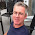Thursday, December 6, 2012

Proportional Reasoning with Percents

I am a big fan of estimation, probably because I am lazy. I think that most percent problems (obviously not all) can be done with just basic numerical / proportional reasoning.

For example, my Grade 7s are pretty darned good by now at doing something like "find 15% of 6.4". They can articulate that 10% of 6.4 is 0.64, and then half of that is 5% = 0.32, so 15% is 0.64 + 0.32 = 0.96 . They can also do something like "find 12% of 88" by reasoning that 10% is 8.8, and then 1% is 0.88, so 12% is 8.8 + 0.88 + 0.88 = 8.8 + 1.76 = 10.56 . Recently I looked over the big semester test that we had given last year in December, and realized that we won't have time this year before the semester exam to cover enough of proportional reasoning to do a problem like "9 is 15% of what number?" using a setup of proportions and of solving by cross multiplication. (We've only just started proportional reasoning this week.) So, today I just decided to throw up some of those types of questions on the board as the Do Now, and the kids were surprisingly clever at figuring it out! One of them said, "It's not that hard. It's like playing Math Detective."

"3 is 10% of what number?" --> This was the first "backwards" type of question I had put on the board, and the kids thought it was quite easy. You're just multipling by 10 times to get to 100%, which is 30.

"12 is 15% of what number?" --> Some kids naturally, intuitively, broke it down to 5% being 4, which is a "nice" percentage to have/know because then you know that 10% is 8, and therefore 100% is 80.

"6 is 2% of what number?" --> To do this, they were also clever. Some did it as 1% is 3, so 100% must be 300 if you know that one out of the 100 parts is 3. One kid immediately thought that you can scale both values up by 50 times in order to reach 100%, to save us from doing two steps of work.

I really liked these discussions, because I think -- in lack of a formal proportional setup -- this is the most intuitive way of approaching percentages, in my mind. It reinforces the meaning of percents and frees kids from fear of these fairly basic everyday concepts.

We discussed briefly why this is proportional reasoning. It tied very nicely to the word problems we did today on proportions, but I am not going to touch cross multiplication until January. (It's just too much, too soon for many of them, and they have already worked very hard to fill in the gaps this semester.)

So, go seventh-graders! I feel very hopeful that, despite my concerns about many of them at the start of the year, the majority of the class is nearly caught up to where they ought to be at this time of the year.

1.Hi Mimi,
What a great post! Your students are very fortunate to have you as their teacher. In my opinion you are setting them up for great success in future math courses.

2.I love this, Mimi! It's SO SO IMPORTANT that we have kids do this! I ask kids for an estimate or even a very approximate answer (just a range is fine) before they can pick up their pencil. Your example of "find 12% of 88" would mean about 10% of 90, so the answer is around 9. Then they can do the break apart like you're doing. This has seriously cut down on the number of "ridiculous" answers that I used to get. Go teachers!

3.Hi Mimi, I love this too. I teach (and do!) the mental percent calculation you described and it's so handy. I'm wondering why cross multiplication is necessary if they already can figure out these problems without it. Are you just teaching it as a "here's a cool thing to know" and another strategy to try?

4.Not necessary for nice percent problems, but is necessary for things like "7 is 1.1% of what number?" (I suppose you can say 7 divided by 1.1 gives you 1%, then times 100 gives you 100%. So 700/1.1 = 7000/11 and you can bypass the cross multiplication, but I'm not sure how many of my 7th-graders would be able to consistently get that type of reasoning correct. Versus I think the proportional setup is easier:
7/x = 1.1%/100% and they can just solve using cross-multiplication."

Anyway, I do teach cross-multiplication in grade 7 as a general skill/tool for solving proportions. I like cross multiplication b/c down the road when they solve things like cos(32) = 2/y, it makes it easy/consistently accurate for them if they just get used to always setting it up as cos(32)/1 = 2/y and solving using cross multiplication. Else some kids can get confused when the variable is in the denominator and they'd randomly give incorrect answers like y = 2cos(32).